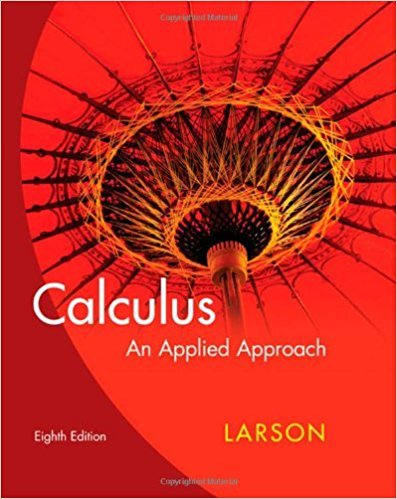×
×

# Solutions for Chapter 5: Integration and Its Applications## Full solutions for Calculus: An Applied Approach | 8th Edition

ISBN: 9780618958252Solutions for Chapter 5: Integration and Its Applications

Solutions for Chapter 5
4 5 0 413 Reviews
19
0
##### ISBN: 9780618958252

Since 102 problems in chapter 5: Integration and Its Applications have been answered, more than 21900 students have viewed full step-by-step solutions from this chapter. Calculus: An Applied Approach was written by and is associated to the ISBN: 9780618958252. Chapter 5: Integration and Its Applications includes 102 full step-by-step solutions. This expansive textbook survival guide covers the following chapters and their solutions. This textbook survival guide was created for the textbook: Calculus: An Applied Approach , edition: 8.

Key Calculus Terms and definitions covered in this textbook
• Algebraic expression

A combination of variables and constants involving addition, subtraction, multiplication, division, powers, and roots

• Argument of a complex number

The argument of a + bi is the direction angle of the vector {a,b}.

• Completing the square

A method of adding a constant to an expression in order to form a perfect square

• De Moivre’s theorem

(r(cos ? + i sin ?))n = r n (cos n? + i sin n?)

• Empty set

A set with no elements

• Equilibrium price

See Equilibrium point.

• General form (of a line)

Ax + By + C = 0, where A and B are not both zero.

• Identity matrix

A square matrix with 1’s in the main diagonal and 0’s elsewhere, p. 534.

• Maximum r-value

The value of |r| at the point on the graph of a polar equation that has the maximum distance from the pole

• Probability function

A function P that assigns a real number to each outcome O in a sample space satisfying: 0 … P1O2 … 1, P12 = 0, and the sum of the probabilities of all outcomes is 1.

• Probability simulation

A numerical simulation of a probability experiment in which assigned numbers appear with the same probabilities as the outcomes of the experiment.

• Relevant domain

The portion of the domain applicable to the situation being modeled.

• Remainder theorem

If a polynomial f(x) is divided by x - c , the remainder is ƒ(c)

• Sinusoid

A function that can be written in the form f(x) = a sin (b (x - h)) + k or f(x) = a cos (b(x - h)) + k. The number a is the amplitude, and the number h is the phase shift.

• Solution of a system in two variables

An ordered pair of real numbers that satisfies all of the equations or inequalities in the system

• Standard form of a polynomial function

ƒ(x) = an x n + an-1x n-1 + Á + a1x + a0

• Transitive property

If a = b and b = c , then a = c. Similar properties hold for the inequality symbols <, >, ?, ?.

• Unit vector in the direction of a vector

A unit vector that has the same direction as the given vector.

• y-intercept

A point that lies on both the graph and the y-axis.

• Zero factor property

If ab = 0 , then either a = 0 or b = 0.

×